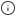# Percentile approximation

In general, percentiles are useful for understanding the distribution of data. The fiftieth percentile is the point at which half of your data is greater and half is lesser. The tenth percentile is the point at which 90% of the data is greater, and 10% is lesser. The ninety-ninth percentile is the point at which 1% is greater, and 99% is lesser.

The fiftieth percentile, or median, is often a more useful measure than the average, especially when your data contains outliers. Outliers can dramatically change the average, but do not affect the median as much. For example, if you have three rooms in your house and two of them are 40℉ (4℃) and one is 130℉ (54℃), the average room temperature is 70℉ (21℃), which doesn't tell you much. However, the fiftieth percentile temperature is 40℉ (4℃), which tells you that at least half your rooms are at refrigerator temperatures (also, you should probably get your heating checked!)

Percentiles are sometimes avoided because calculating them requires more CPU and memory than an average or other aggregate measures. This is because an exact computation of the percentile needs the full dataset as an ordered list. Timescale uses approximation algorithms to calculate a percentile without requiring all of the data. This also makes them more compatible with continuous aggregates. By default, TimescaleDB uses `uddsketch`, but you can also choose to use `tdigest`. For more information about these algorithms, see the advanced aggregation methods documentation.###### Note

Technically, a percentile divides a group into 100 equally sized pieces, while a quantile divides a group into an arbitrary number of pieces. Because we don't always use exactly 100 buckets, "quantile" is the more technically correct term in this case. However, we use the word "percentile" because it's a more common word for this type of function.

Keywords